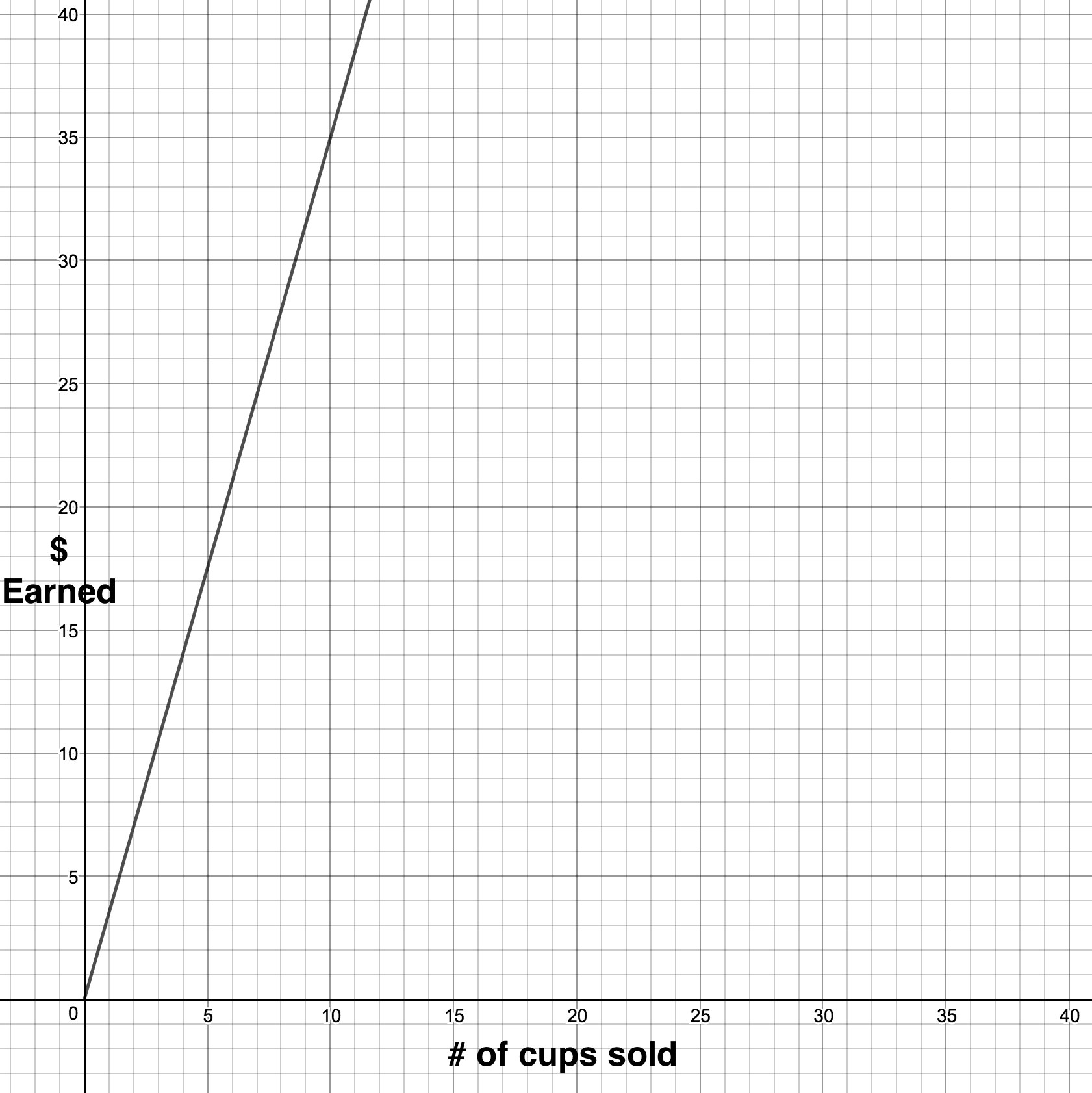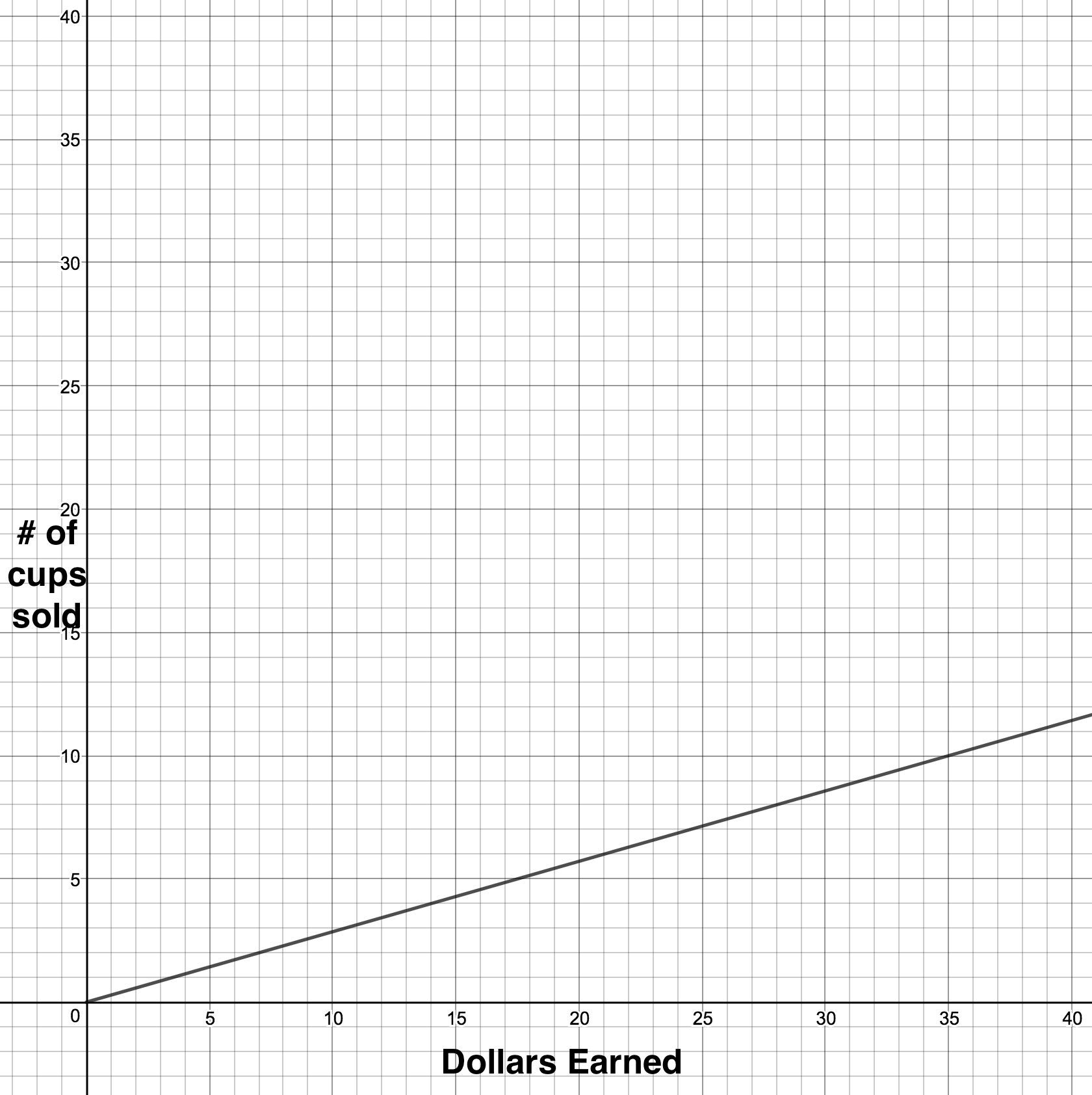# Linear Equations, Inequalities and Systems

## Objective

Find inverse functions algebraically, and model inverse functions from contextual situations.

## Common Core Standards

### Core Standards

?

• F.BF.B.4.A — Solve an equation of the form f(x) = c for a simple function f that has an inverse and write an expression for the inverse. For example, f(x) =2 x3 or f(x) = (x+1)/(x—1) for x ? 1.

• A.CED.A.4 — Rearrange formulas to highlight a quantity of interest, using the same reasoning as in solving equations. For example, rearrange Ohm's law V = IR to highlight resistance R.

• F.IF.B.6 — Calculate and interpret the average rate of change of a function (presented symbolically or as a table) over a specified interval. Estimate the rate of change from a graph. Modeling is best interpreted not as a collection of isolated topics but in relation to other standards. Making mathematical models is a Standard for Mathematical Practice, and specific modeling standards appear throughout the high school standards indicated by a star symbol (★). The star symbol sometimes appears on the heading for a group of standards; in that case, it should be understood to apply to all standards in that group.

?

• 8.F.A.1

• 8.F.B.4

## Criteria for Success

?

1. Algebraically manipulate a linear equation so that the equation is solved for $x$ instead of $y$
2. Describe why it is necessary to switch the variables in the equation when algebraically determining the inverse of a linear equation.
3. Compare the rates of change in a function and its inverse.
4. Use algebraic manipulation to model the inverse of a function, and describe how the independent and dependent variables are affected.

## Anchor Problems

?

### Problem 1

Below is a function and its inverse from the previous lesson.Write the equations that model each of these functions. How do you calculate the inverse algebraically?

### Problem 2

Find the algebraic inverse of the following function:

${g(x)=3x -4}$

## Problem Set

?

The following resources include problems and activities aligned to the objective of the lesson that can be used to create your own problem set.

• Include problems where students are given the inverse function and need to find the original function.
• Include problems where students are given the function graphically and are asked to determine the inverse algebraically.
• Include problems where two functions are given and students are asked to determine if they are inverse of one another, using any method they choose (algebraic manipulation, table of values, or graphically).

?

### Problem 1

What is an equation of the line formed when the line ${y=3x+1}$ is reflected in the line ${y=x}$?

a.     ${y=3x-1}$

b.     ${y=\frac{x-1}{3}}$

c.     ${y= \frac{x}{3}-1}$

d.     ${x=y}$

Question #10

### Problem 2

What is the inverse of the function ${y=2x+3}$

a.     ${x=\frac{1}{2}y-\frac{3}{2}}$

b.     ${x=\frac{1}{2}x-\frac{3}{2}}$

c.     ${y=2x-3}$

d.     ${x=-2y-3}$

Question #11